#### A polyhedral C-space obstacle

Most of the previous ideas generalize nicely for the case of a polyhedral robot that is capable of translation only in a 3D world that contains polyhedral obstacles. If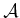and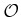are convex polyhedra, the resulting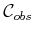is a convex polyhedron.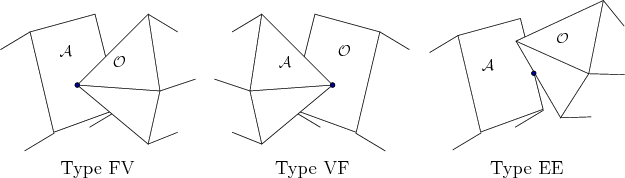There are three different kinds of contacts that each lead to half-spaces in:

1. Type FV: A face ofand a vertex of2. Type VF: A vertex ofand a face of3. Type EE: An edge ofand an edge of.
These are shown in Figure 4.20. Each half-space defines a face of the polyhedron,. The representation ofcan be constructed in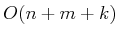time, in whichis the number of faces of,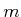is the number of faces of, andis the number of faces of, which is at most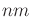.

Steven M LaValle 2020-08-14